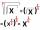# Bomber

Bomber flies 10 km at 600 km/h. At what horizontal distance from the target, must pilot drop the bomb to hit the target?

Don't care about air resistance and consider the gravitational acceleration g=9.81 m/s2.

Result

The pilot must drop the bomb on target before:  7525 m

#### Solution:Leave us a comment of example and its solution (i.e. if it is still somewhat unclear...):

Showing 0 comments:Be the first to comment!#### To solve this example are needed these knowledge from mathematics:

Looking for help with calculating roots of a quadratic equation? Do you want to convert length units?

## Next similar examples:

1. Theorem proveWe want to prove the sentense: If the natural number n is divisible by six, then n is divisible by three. From what assumption we started?
2. FractionFor what x expression ? equals zero?
3. Square rootsWhat is equal to the product of the square roots of 295936?
4. ProductThe product of two consecutive odd numbers is 8463. What are this numbers?
5. AlgebraX+y=5, find xy (find the product of x and y if x+y = 5)
6. EquationEquation ? has one root x1 = 8. Determine the coefficient b and the second root x2.
7. Quadratic equationFind the roots of the quadratic equation: 3x2-4x + (-4) = 0.
8. DiscriminantDetermine the discriminant of the equation: ?
9. RootsDetermine the quadratic equation absolute coefficient q, that the equation has a real double root and the root x calculate: ?
10. EQ2Solve quadratic equation: ?
11. Eq with reciprocalSolve given equation with reciprocal member: a-6/a+10=4/8
12. Functions f,gFind g(1) if g(x) = 3x - x2 Find f(5) if f(x) = x + 1/2
13. Quadratic equationSolve quadratic equation: 2x2-58x+396=0
14. 6 termsFind the first six terms of the sequence. a1 = 7, an = an-1 + 6
15. Quadratic equationQuadratic equation ? has roots x1 = 80 and x2 = 78. Calculate the coefficients b and c.
16. Volleyball8 girls wants to play volleyball against boys. On the field at one time can be six players per team. How many initial teams of this girls may trainer to choose?
17. Nineteenth memberFind the nineteenth member of the arithmetic sequence: a1=33 d=5 find a19